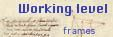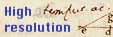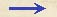Overview Level of Folio 100 vSize Height 193 mm, width 135 mm. Watermark No watermark could be identified by the project group. Drake's identification: Watermark type 25. Drake's description: Siren in 39mm circle, crown above. Watermark found in 1632. Comments Contains calculations, drawings, minimal text. Relation to the Discorsi: related to 3/13-pr-06.
 Folio 100 v (final text) 1 al quantunque C01 G. 32 8989 = 4494 + 4495 4494 * 4495 = 22470 + 40446 + + 17976 + 17976 = 20200630 25 - 20200630 = [?] sqrt 24802264 = 4980 5000 - 4980 = 20 C02 25 - 20200530 = 479960 sqrt 4799460 = 2190 5000 - 2190 = 2810 C03 (22 * 22) : 12 22 * 22 = 484 484 : 12 = 40 C04 1 + 4 + 9 + 16 = 30 64_30_15_8 64 * 8 = 512 30 * 15 = 450Overview Level of Folio 100 v